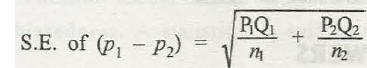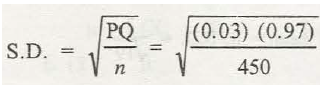Let’s assume that the proportion of defectives is P and a random sample of size n, which is large enough, is drawn.  Then the sampling distribution of P is almost a normal distribution with a mean= P and standard deviation= S.E of sample proportion =  where Q= 1-P.When the random sample is drawn from a finite population, without replacement, then the Standard deviation formula needs to be multiplied with a correction factor

If p₁ and p₂ are individual proportions taken from individual samples of size n₁ and n₂ drawn from a population, then,

S.E of (p₁- p₂) =  where P₁+Q₁=1 and P₂+Q₂=1

Example:

If 3% of the tools produced by a certain machine are defective, then what is the probability that in a shipment of 450 such tools, 2% or more will be defective?

Solution:

n= 450, p=3%= 0.03S.D=

= = 0.008

Therefore, probability= P [p > 0.02]

= P [z > -1.25] = 0.5 + φ (1.25)

= 0.5 + 0.3944 = 0.8944.

Numerical problems:

1. When we take a sample from an infinite population, what happens to standard error of mean if sample size is (a) increased from 30 to 270, (b) decreased from 256 to 16?
2. The number of letters that a department receives each day can be modelled by a distribution having mean 25 and standard deviation 4. For a random sample of 30 days, what will be the probability that the sample mean will be less than 26?
3. From a population of large number of men with a S.D. 5, a sample is drawn and the standard error is found to be 0.5, what is the sample size?
4. A machine produces a component for a transistor set of the total produce, 6 % are defective. A random sample of 5 components is taken for examination from (i) a very large lot of produce, a box of 10 components. Find the mean and S.D. of the average number of defectives found among the 5 components taken for examination.
5. A population consists of 5 numbers (2, 3, 6, 8, and 11). Consider all possible samples of size two which can be drawn with replacement from this population. Calculate the S.E. of sample means.
6. A random sample of size 400 is taken from an infinite population having the mean µ = 86 and the variance of σ2 = 625. What is the probability that X will be greater than 90?
7. A random sample of 400 mangoes was taken from a large consignment and 30 were found to be bad. Find the S.E. of the population of bad ones in a sample of this size.
8. A population consists of five numbers 2, 3, 6, 8, 11. Consider all possible samples of size two which can be drawn without replacement from the population. Find

(a)   Mean of the population

(b)   The Standard deviation of the population

(c)   Mean of sampling distribution of means

(d)   Standard deviation of the sampling distribution of means.

1. A population consists of 20 elements, has mean 9 and S.D. 3 and a sample of 5 elements is taken without replacement. Find the mean and S.D. of the sampling distribution of the mean. What will be the S.D. for samples of size 10?

Solutions:

1.(a) It is divided by 3

(b) It is multiplied by 4

1. 0.9147
2. 100
3. Mean= 0.06, S.D= 0.106
4. 2.32
5. 0.0007
6. 0.013
7. (a) 6, (b) 3.29, (c) 6, (d) 2.12.
8. For sample of 5 elements, sampling mean = 8, S.D. =

For sample of 10 elements, sampling mean = 8, S.D. =### Customer Reviews

My Homework Help
Rated 5.0 out of 5 based on 510 customer reviews at